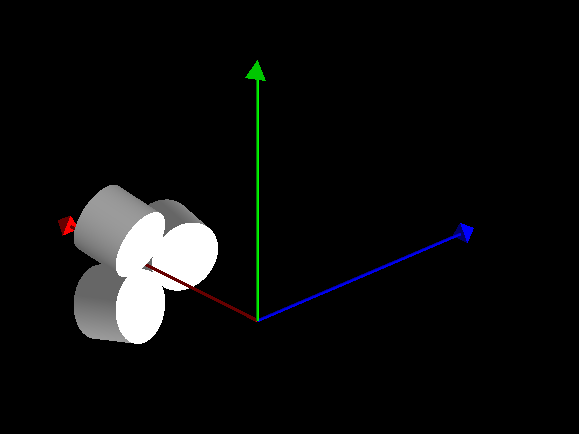# Placing a detector with 6 degrees of freedom

Good day experts,

I need to place a detector by specifying it’s x, y, z, pitch, yaw and roll. Could you please advise on how to do this.

Thank you
Aliyu

`G4RotationMatrix` (it’s an alias to `CLHEP::HepRotation`) has a constructor with three Euler angles.

Thank you very much @evc. I used your response to search on the examples that implemented Euler angle and I cam across the transform example under geometry.

Is this what you are referring to?

Thank you
Aliyu

I recommend you to use `G4PVPlacement` with `G4Transform3D`. An object of `G4Transform3D` class can be constructed as a sequence of simple transformations. For example:

``````G4Transform3D T = G4RotateZ3D(yaw)*G4RotateY3D(pitch)*G4RotateX3D(roll);
``````

For explanation, please see wiki , the section General rotations

Good morning @evc,

Thank you again and I sincerely apologize for my basic knowledge. So those that mean I have to define pitch, yaw and roll as 3x3 matrices?

G4ThreeVector Col_pos = G4ThreeVector(Col_xpos, Col_ypos, Col_zpos);

G4double Col_yaw = 0.*deg;
G4double Col_pitch= 0.*deg;
G4double Col_roll = 0.*deg;

G4Transform3D transform1 = G4RotateZ3D(Col_yaw)*G4RotateY3D(Col_pitch)*G4RotateX3D(Col_roll);

G4VPhysicalVolume* shield_phys = new G4PVPlacement(transform2, Col_pos, shield_log, “Shield2”, sludge_log, true, 0, checkOverlaps);

G4Placement gave error which implies that I can not transform as well as translate. How then do I decide the x, y, and z position of my collimator?

A more detailed example of G4Placement will be appreciated.

Thank you
Aliyu

you either use the constructor for `G4PVPlacement` with a rotation matrix and a translation vector,
or as @evc suggested a single `G4Transform3D`.

You can check the header for these constructors: geant4/source/geometry/volumes/include/G4PVPlacement.hh at master · Geant4/geant4 · GitHub

You could simply add `G4Translate3D(G4ThreeVector(Col_xpos, Col_ypos, Col_zpos))` (or `G4TranslateX3D(Col_xpos)*G4TranslateY3D(Col_ypos)*G4TranslateZ3D(Col_zpos)...`) to your transformation at the correct position (first translate then rotate or vice versa?) and should be good to goThank you very much for response sir. So my confusion now is, how do I specify theta, phi or psi of the collimator assuming I define my G4placement as follows:

G4VPhysicalVolume* shield_phys = new G4PVPlacement(G4Translate3D(G4ThreeVector(Col_xpos, Col_ypos, Col_zpos)), shield_log, “Shield2”, sludge_log, true, 0, checkOverlaps);

Aliyu

An object of `G4Transform3D` class can be built as sequence of simple rotations and translations. Please see the topic

that contains a relevant example.

@evc If my detector positions are like ,

theta = 180.000000deg;
phi= 180.000000
deg;

theta[ 1] = 142.622561deg;
phi[ 1] = 359.999847
deg;

theta[ 2] = 142.622528deg;
phi[ 2] = 71.999866
deg;

then how to proceed?

for this case loop won’t work, right?

Is this three alternative positions of the detector, that should be compared?

or

the positions that the detector may take between runs?

or

the positions of different parts of the detector?

@evc These are the three alternative positions of the detector. I like to place many such detector , like 100.
Theta Phi
142.6 0.0
142.6 72.0
142.6 144.0
142.6 216.0
142.6 288.0
116.6 324.0
116.6 36.0
116.6 108.0
116.6 180.0

Assuming that you have N detectors placed at different `theta` and `phi`, but at the same `distance` from the origin, then the transformation of a detector can be done in three steps:

1. Shift along Z by `distance`
2. Rotation around Y by `theta[i]`
3. Rotation around Z by `phi[i]`
``````  G4Translate3D shiftZ(0, 0, distance);
for (G4int i = 0; i < N; ++i)
{
G4int copyNumber = i;
G4RotateY3D rotTheta(theta[i]);
G4RotateZ3D rotPhi(phi[i]);
auto transform = rotPhi*rotTheta*shiftZ;
new G4PVPlacement(transform, logicDet, "Detector", logicWorld,
false, copyNumber, checkOverlaps);
}
``````

Yes! @evc thank you so much .

Does G4RotateY3D rotTheta(theta[i]); G4RotateZ3D rotPhi(phi[i]); here relates to spherical polar coordinates , like r * [ sin(theta) * cos(phi), sin(theta) * sin(phi) ], cos(theta)) ??

For placements like
theta= 75deg;
phi= 0
deg;

theta= 75deg;
phi= 120
deg;

theta= 75deg;
phi= 240
deg;

I changed two of the line
G4RotateY3D rotTheta(theta[i]);
G4RotateZ3D rotPhi(phi[i]);
to
G4RotateY3D rotTheta(theta[i]);
G4RotateX3D rotPhi(phi[i]);
I got like position along x axis but I want along Y axis. What to do?Yes, `r, theta and ph`i specify the position of a point in the spherical coordinates, and your expression for calculation of the position of the point in the cartesian coordinates is correct.

1 Like

@evc But How can I modify the conventional spherical coordinate? Suppose I want to use sin(theta) * sin(phi),sin(theta) * cos(phi) , - cos(theta) as my X,Y and Z .

Try to think in terms of rotation. For example, to change X → Y, Y → Z, Z → X you need to perform a rotation around `vector(1,1,1)` by `120` degrees:

`G4Rotate3D(120*deg, G4ThreeVector(1,1,1))`

To change X → Y, Y → – X apply rotation around Z by 90 deg.

1 Like

@evc I could think of like this way,
If I use coordinates like X= r * cos(theta)cos(phi) , Y = -r * sin(phi), Z = r * sin(theta)cos(phi) then my problem would be solved.
Then I can place those three detector along Y axis also. Basically I want the rings around +ve Y and -ve Y axis.

It can be done in different ways. For example, you place your detectors around Z, and then rotate them by – 90 degrees around X, see the attachment.
Another option is to rotate around X first, and then make manipulations around Y, instead of Z, see commented code in the attachment.

DetectorConstruction.cc (3.6 KB)

1 Like

Dear @evc,

Apologies for my basic understanding. Please is there any example you can point me to that record theta, phi and psi via SteppingAction?

Thank you
Aliyu

In SteppingAction you have the `copyNumber` of the detector:

``````    new G4PVPlacement(transform, logicDet, "Detector", logicWorld,
false, copyNumber, checkOverlaps);
``````

The `copyNumber` identifies the detector where current position is, so you can get all information about the detector.